# Electromagnetic Induction | Faraday Law & Lenz’s Law Explained

To best understand the Electromagnetic Induction lets first have a look on two important laws relating to the subject of this article. Lenz’s law is named after a Russian physicist of Baltic German descent Heinrich Lenz in 1834, and it states that, if an induced current flows, its direction is always such that it will oppose the change which produced it.

Lenz’s law is shown by the negative sign in Faraday’s law of inductionwhich indicates that the induced voltage () and the change in magnetic flux () have opposite signs. It is a qualitative law that specifies the direction of induced current but saeys nothing about its magnitude. Lenz’s Law explains the direction of many effects in electromagnetism, such as the direction of voltage induced in an inductor or wire loop by a changing current, or why eddy currents exert a drag force on moving objects in a magnetic field.

Electromagnetic Induction: The phenomenon by which an emf is induced in a circuit (and hence current flows when the circuit is closed) when magnetic flux linking with it changes is called electromagnetic induction.

Fleming Right Hand rule is used to demonstrate the direction of the emf or direction of Induced Emf also termed as Generator Action in Electromagnetic Induction.

This rule states “Hold out the right hand with the first finger, second finger and thumb at right angles to each other. If forefinger represents the direction of the line of force, the thumb points in the direction of motion or applied force, then second finger points in the direction of the induced current.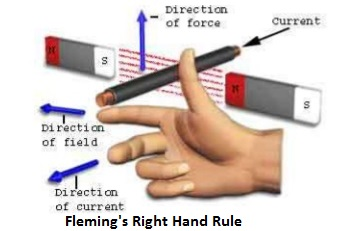Fleming Left Hand rule is used to demonstrate the direction of the emf force developed also termed as Motor Action.

It is found that whenever a current carrying conductor is placed inside a magnetic field, a force acts on the conductor, in a direction, perpendicular both to the direction of the electric current and the magnetic field.

Hold out your left hand with forefinger, second finger and thumb at right angle to one another. If the fore finger represents the direction of the field and the second finger that of the current, then thumb gives the direction of the force.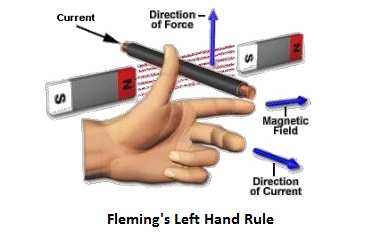## Lenz’s Law of Electromagnetic Induction

Let’s understand the Lenz’s law by below given two definitions and then we shall see few examples to understand the concept of Electromagnetic Induction in more simple way so that it is easy for new students to absorb the complexity of the concept.

Lenz’s Law Definition 1: This just says that ‘the direction of the Induced Current is such that it opposes the change that causes it.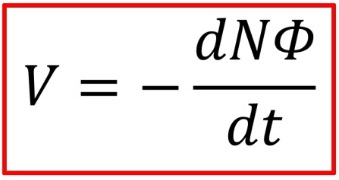If you push a wire through a field the induced current makes a force that pushes back.

If a field is pointing one way and a conductor moves through it, then the induced current makes a field that points the opposite way

### Lenz’s Law Definition 2:

Faraday’s law gives the magnitude and direction of the induced emf, and therefore the direction of any induced current. Lenz’s law is a simple way to get the directions straight, with less effort. Lenz’s Law states that induced emf is directed so that any induced current flow will oppose the change in magnetic flux (which causes the induced emf). This is easier to use than to say:

Decreasing magnetic flux ===> emf creates additional magnetic field

Increasing flux ===> emf creates opposed magnetic field

### Lenz’s Law Definition 3:

When an emf is generated by a change in magnetic flux according to Faraday’s Law, the polarity of the induced emf is such that it produces a current whose magnetic field opposes the change which produces it. The induced magnetic field inside any loop of wire always acts to keep the magnetic flux in the loop constant. In the examples below, if the B field is increasing, the induced field acts in opposition to it. If it is decreasing, the induced field acts in the direction of the applied field to try to keep it constant.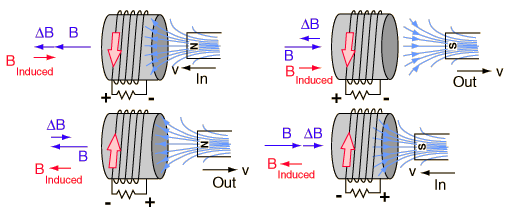Definition in terms of Magnet in Copper Pipe:

As per Faraday’s Law, as the magnet falls through the copper pipe there is a changing Flux on the copper. This induces eddy currents in the copper pipe. As per Lenz’s Law, the eddy currents flow in such a way that they create a magnetic field which opposes the change that produced them.

Definition in terms of Induction Braking / Eddy current braking

As per Faraday’s Law, as the magnet is brought near to the rotating disc there is a changing Flux on any point in the copper. This induces eddy currents in the copper disc. As per Lenz’s Law, the eddy currents flow in such a way that they create a magnetic field which opposes the change that produced them.

### Examples of Lenz’s Law Implementation

Lenz Law Case Study / Example 1: When a magnet is moving towards the coil.

• Flux is increasing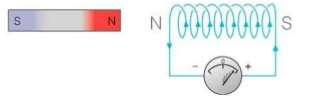• Current will be produced in such a direction so as to oppose the increase in flux

The magnetic field created will oppose its own cause or we can say opposes the increase in flux through the coil. This is possible only if approaching coil side attains north polarity, as we know similar poles repel each other.

#### Lenz Law Case Study / Example 2:When a magnet is moving away from the coil

• Flux linking with coil decreases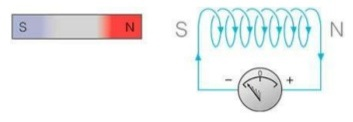• an emf and hence current is induced in the coil and this current will creates its own magnetic field

According to Lenz’s law, this magnetic field created will oppose its own cause or we can say opposes the decrease in flux through the coil and this is possible only if approaching coil side attains south polarity, as we know dissimilar poles attract each other.

Now look at below animation and comment what is this and that this is correct or not: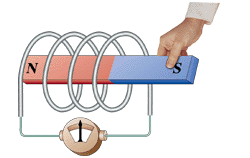error: Content is protected !!